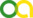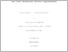Bohm, Marvin (2019). An entropy stable nodal discontinuous Galerkin method for the resistive MHD equations. PhD thesis, Universität zu Köln.Preview
PDF
Dissertation.pdf

## Abstract

We present a discretely entropy stable discontinuous Galerkin (DG) method for the resistive magneto-hydrodynamic (MHD) equations on three-dimensional curvilinear un- structured hexahedral meshes. Compared to other fluid dynamics systems such as the shallow water equations or the compressible Navier-Stokes equations, the resistive MHD equations need special considerations because of the divergence-free constraint on the magnetic field. For instance, it is well known that for the symmetrization of the ideal MHD system as well as the continuous entropy analysis a non-conservative term propor- tional to the divergence of the magnetic field, typically referred to as the Powell term, must be included. As a consequence, the mimicry of the continuous entropy analysis in the discrete sense demands a suitable DG approximation of the non-conservative terms in addition to the ideal MHD terms. We focus on the resistive MHD equations. Subsequently, our first result is a proof that the resistive terms are symmetric and positive-definite when formulated in entropy space as gradients of the entropy variables. This enables us to show that the entropy inequality holds for the resistive MHD equations. The continuous analysis is the key for our DG discretization and guides the path for the construction of an approximation that discretely mimics the entropy inequality, typically termed entropy stability. The discrete analysis relies on the summation-by-parts (SBP) property, which is satisfied by the DG spectral element method (DGSEM) with Legendre-Gauss-Lobatto (LGL) nodes. With the help of a resulting split form approximation and by incorporating specific dicretiza- tions of the non-conservative terms, we obtain an overall entropy conservative DG scheme for the ideal MHD equations. We extend the scheme to an entropy stable approximation by adding appropriate dissipation terms. Further, we provide a detailed derivation and analysis of the entropy stable discretization on three-dimensional curvilinear meshes. Although the divergence-free constraint is included in the non-conservative terms, the resulting method has no particular treatment to control the magnetic field divergence errors, which pollute the solution quality. Hence, we also extend the standard resistive MHD equations and the according DG approximation with a divergence cleaning mech- anism that is based on a generalized Lagrange multiplier (GLM). Moreover, we equip the resulting scheme with certain shock capturing methods in order to regularize the approximation in oscillatory regions close to discontinuities. We provide numerical examples that verify the theoretical properties of the entropy stable method. Also, we demonstrate the increased robustness of the entropy stable method with a series of challenging numerical results, before we finally apply it to a real space physics model describing atmospheric plasma interactions.

Item Type: Thesis (PhD thesis)
Creators:
CreatorsEmailORCIDORCID Put Code
Bohm, MarvinUNSPECIFIEDUNSPECIFIEDUNSPECIFIED
URN: urn:nbn:de:hbz:38-94356
Date: 14 March 2019
Language: English
Faculty: Faculty of Mathematics and Natural Sciences
Divisions: Faculty of Mathematics and Natural Sciences > Department of Mathematics and Computer Science > Mathematical Institute
Subjects: Mathematics
Physics
Uncontrolled Keywords:
KeywordsLanguage
Discontinuous GalerkinEnglish
MagnetohydrodynamicsEnglish
Date of oral exam: 15 February 2019
Referee:
Gassner, GregorProf. Dr.
Klingenberg, ChristianProf. Dr.
Refereed: Yes
URI: http://kups.ub.uni-koeln.de/id/eprint/9435View Item